# Perplexity

In information theory, perplexity is a measurement of how well a probability distribution or probability model predicts a sample. It may be used to compare probability models. A low perplexity indicates the probability distribution is good at predicting the sample.

## Perplexity of a probability distribution

The perplexity PP of a discrete probability distribution p is defined as

${\mathit {PP}}(p):=2^{H(p)}=2^{-\sum _{x}p(x)\log _{2}p(x)}=\prod _{x}p(x)^{-p(x)}$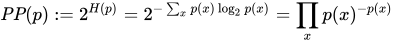where H(p) is the entropy (in bits) of the distribution and x ranges over events. (The base need not be 2: The perplexity is independent of the base, provided that the entropy and the exponentiation use the same base.) This measure is also known in some domains as the (order-1 true) diversity.

Perplexity of a random variable X may be defined as the perplexity of the distribution over its possible values x.

In the special case where p models a fair k-sided die (a uniform distribution over k discrete events), its perplexity is k. A random variable with perplexity k has the same uncertainty as a fair k-sided die, and one is said to be "k-ways perplexed" about the value of the random variable. (Unless it is a fair k-sided die, more than k values will be possible, but the overall uncertainty is no greater because some of these values will have probability greater than 1/k, decreasing the overall value while summing.)

Perplexity is sometimes used as a measure of how hard a prediction problem is. This is not always accurate. If you have two choices, one with probability 0.9, then your chances of a correct guess are 90 percent using the optimal strategy. The perplexity is 2−0.9 log2 0.9 - 0.1 log2 0.1= 1.38. The inverse of the perplexity (which, in the case of the fair k-sided die, represents the probability of guessing correctly), is 1/1.38 = 0.72, not 0.9.

The perplexity is the exponentiation of the entropy, which is a more clearcut quantity. The entropy is a measure of the expected, or "average", number of bits required to encode the outcome of the random variable, using a theoretical optimal variable-length code, e.g. It can equivalently be regarded as the expected information gain from learning the outcome of the random variable.

## Perplexity of a probability model

A model of an unknown probability distribution p, may be proposed based on a training sample that was drawn from p. Given a proposed probability model q, one may evaluate q by asking how well it predicts a separate test sample x1, x2, ..., xN also drawn from p. The perplexity of the model q is defined as

$b^{-{\frac {1}{N}}\sum _{i=1}^{N}\log _{b}q(x_{i})}$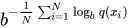where $b$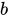is customarily 2. Better models q of the unknown distribution p will tend to assign higher probabilities q(xi) to the test events. Thus, they have lower perplexity: they are less surprised by the test sample.

The exponent above may be regarded as the average number of bits needed to represent a test event xi if one uses an optimal code based on q. Low-perplexity models do a better job of compressing the test sample, requiring few bits per test element on average because q(xi) tends to be high.

The exponent may also be regarded as a cross-entropy,

$H({\tilde {p}},q)=-\sum _{x}{\tilde {p}}(x)\log _{2}q(x)$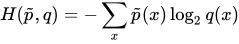where ${\tilde {p}}$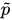denotes the empirical distribution of the test sample (i.e., ${\tilde {p}}(x)=n/N$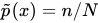if x appeared n times in the test sample of size N).

## Perplexity per word

In natural language processing, perplexity is a way of evaluating language models. A language model is a probability distribution over entire sentences or texts.

Using the definition of perplexity for a probability model, one might find, for example, that the average sentence xi in the test sample could be coded in 190 bits (i.e., the test sentences had an average log-probability of -190). This would give an enormous model perplexity of 2190 per sentence. However, it is more common to normalize for sentence length and consider only the number of bits per word. Thus, if the test sample's sentences comprised a total of 1,000 words, and could be coded using a total of 7.95 bits per word, one could report a model perplexity of 27.95 = 247 per word. In other words, the model is as confused on test data as if it had to choose uniformly and independently among 247 possibilities for each word.

The lowest perplexity that has been published on the Brown Corpus (1 million words of American English of varying topics and genres) as of 1992 is indeed about 247 per word, corresponding to a cross-entropy of log2247 = 7.95 bits per word or 1.75 bits per letter using a trigram model. It is often possible to achieve lower perplexity on more specialized corpora, as they are more predictable.

Again, simply guessing that the next word in the Brown corpus is the word "the" will have an accuracy of 7 percent, not 1/247 = 0.4 percent, as a naive use of perplexity as a measure of predictiveness might lead one to believe. This guess is based on the unigram statistics of the Brown corpus, not on the trigram statistics, which yielded the word perplexity 247. Using trigram statistics would further improve the chances of a correct guess.